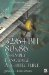Exercises1. Write code for your favorite processor to convert an image 256 pixels wide by 256 pixels high from 32-bit RGB data to 24-bit RGB.

2. Same as problem #1 but convert to 5:6:5-bit RGB (5 bits red, 6 bits green, 5 bits blue).

3. Convert from packed 5:5:5 RGB to 32-bit RGB.

4. For {SSE2} what instruction is needed to convert from a 16-bit signed number to 32-bit? Unsigned?

5. For {SSE2} write code snippets to pack four 128-bit vectors from packed 32-bit to packed 8-bit.

6. Write a function to convert a 128-bit vector of 32-bit packed integers from big-endian to little-endian and vice versa.

7. Same as problem #6 but 32-bit single-precision floating-point values.

8. Same as problem #6 but 64-bit double-precision floating-point values.

9. The beginning of this chapter shows a diagram for an AoS to SoA. Write it using

1. 3DNow!

2. SSE

3. SSE2

10. The beginning of this chapter shows a diagram for an AoS to SoA. Write the reverse from SoA to AoS using

1. 3DNow!

2. SSE

3. SSE232/64-Bit 80x86 Assembly Language Architecture
ISBN: 1598220020
EAN: 2147483647
Year: 2003
Pages: 191

Similar book on Amazon